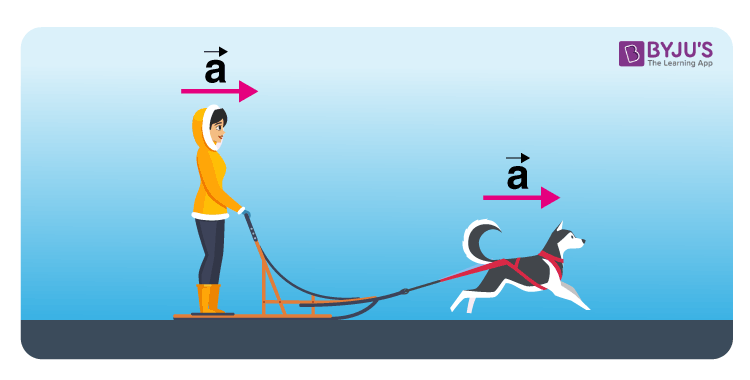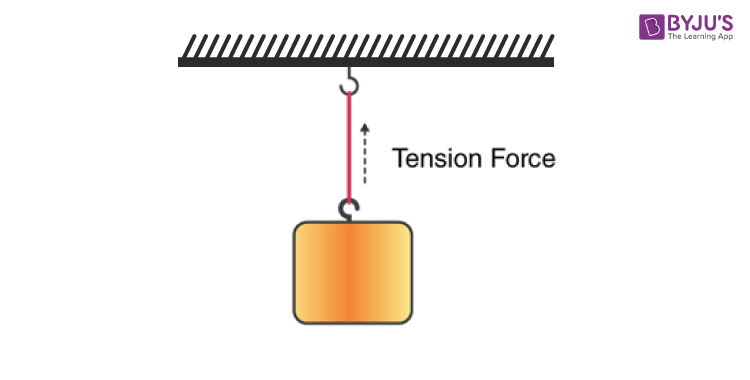# Tension

Two or more physical objects that are in contact, exert forces on each other. Based on the objects in contact we give these contact forces different names. If one of these objects in contact happens to be a string, rope, cable or spring, we call the force as tension.

 Ropes and cables efficiently transfer force over a significant distance. A sledge pulled by a team of Siberian Huskies is a great example to validate this fact.The ropes secured to the sledge lets the dogs run with a larger range of motion as compared to the team of Huskies that are required to push on the back surface of the sledge from behind using the normal force.

## What is Tension?

Tension is a force along the length of a medium, especially a force carried by a flexible medium, such as a rope or cable.

Tension can be defined as an action-reaction pair of forces acting at each end of the said elements. While considering a rope, the tension force is felt by every section of the rope in both the directions, apart from the endpoints. The endpoints experience tension on one side and the force from the weight attached. Throughout the string, the tension varies in some circumstances.

### Common Misconception

Tension is a pulling force and not a pushing force as ropes can’t push effectively. Attempting to push the rope will cause the rope to go slack losing the tension it possesses. This might sound obvious but while illustrating the forces acting on an object, people often draw the force of tension going in the wrong direction. Hence it is important to remember that tension can only pull on an object but not push against it.## How do we calculate the force of tension?

The tension on an object is equal to the product of the mass of the object and gravitational force added to the product of the mass and acceleration. Mathematically, it is represented as follows:
T = mg + ma
Let us look at the solved example to better understand.

1. There is a 10 kg mass hanging from a rope. What is the tension in the rope if the acceleration of the mass is zero?

Solution:
We know that the force of tension is calculated using the formula T = mg + ma.
Substituting the values in the equation, we get T= (10 kg) (9.8 m/s2) + (10 kg) (0)
T = 108 N
(i)Now, assume that there is an acceleration +5 m/s2 upwards. Substituting, we get T= (10 kg) (9.8 m/s2) + (10 kg) (5 m/s2)
T=148 N
(ii) Assume a downwards acceleration of a = -5m/s2
Substituting, we get T= (10 kg) (9.8 m/s2) + (10 kg) (-5)
T= 48 N
Similar Articles:

## Frequently Asked Questions on Tension

Q1

### Why is the work done by tension always zero?

Work done depends on both force and displacement. Tension is a force but it doesn’t cause any displacement. If the work done is given by the following equation:
W = FS
where F is the force and S is the displacement
then, in the case of tension
W = F × 0 = 0
Therefore, the work done by tension is zero.

Q2

### How can we find the direction of the tension force?

The direction of tension is the pull which is given the name tension. Thus, the tension will point away from the mass in the direction of the string/rope. In case of the hanging mass, the string pulls it upwards, so the string/rope exerts an upper force on the mass and the tension will be in the upper side.

Q3

### What is the tension in the spring if two equal forces of 5 N is applied to its end?

The string has a force of tension of 10 N. The spring is stretched by an equal force of 5N in the opposite direction giving rise to a tension of 5+5=10N

Q4

### How does gravity affect the force of tension?

Tension acts in the opposite direction of the gravitational force. If the object is hanging it must be balanced by tension, otherwise, it will accelerate down due to gravity.

Q5

### In what cases would the force of tension between two bodies connected by the same rope differ?

The rope acts as a transferring agent of the force, so there should never be a scenario in which two objects connected by one rope experience tension forces of different magnitudes.

Stay tuned to BYJU’S and Fall in Love with Learning!